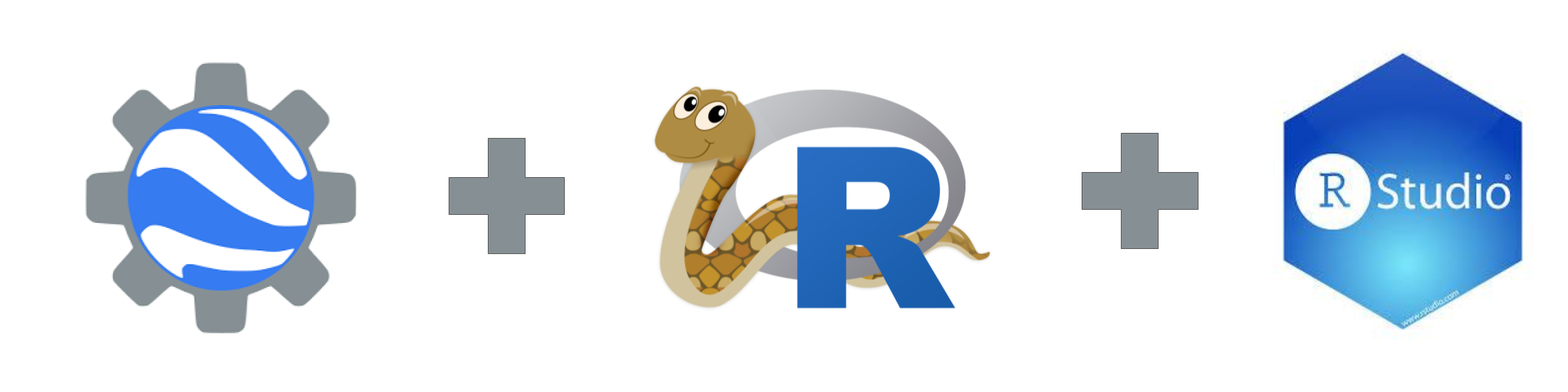A short tutorial on how to use Google Earth Engine inside RStudio with the GEE Python API and `reticulate` - the R interface to Python.To use Google Earth Engine in RStudio we need several ingredients. First of all we need Python to use the Earth Engine Python API in order to send our requests to the Earth Engine servers. Then we need `reticulate`.

`reticulate` allows us to combine Python and R code in RStudio. So, when values are returned from Python to R they are converted back to R types.

# Setup

Where can you get the Earth Engine Python API? Well, there are many installation options, here I use the conda option. The workflow is from Tyler Erickson’s Earth Engine Python API - breakout session from the Geo4Good summit 2019.

### Terminal

Open your Terminal and write (check the conda cheat sheet for Windows & Mac differences):

``````### Terminal

conda create --name gee-demo      # Create a conda environment

conda activate gee-demo           # Activate the environment

conda install -c conda-forge earthengine-api # Install the Earth Engine Python API

earthengine authenticate          # Authenticate your access with the command line tool

conda install pandas
conda install numpy``````

### In RStudio

In RStudio, install the `reticulate` package and apply the `use_condaenv()` function, which enables you to specify which version of Python in the `conda` environment you want to use.

``````### R

install.packages(reticulate)
library(reticulate)

use_condaenv("gee-demo", conda = "auto",required = TRUE)``````

Import the Earth Engine API (ee) and run the `ee\$Initialize` function to trigger the authentication.

``````### R

ee = import("ee")          # Import the Earth Engine library

ee\$Initialize()            # Trigger the authentication

np = import("numpy")       # Import Numpy
pd = import("pandas")      # Import Pandas``````

# Get Sentinel-2 data from Earth Engine

Let’s assume we are interested in a high resolution, cloud free summer scene from a urban green area in Berlin, Germany. Here is the workflow to get the data into R.

``````### R
geometry = ee\$Geometry\$Point(13.481643640792527,52.48959983479137);

S2 = ee\$ImageCollection("COPERNICUS/S2_SR")

# select images between May and August of 2019
S2 = S2\$filterDate(ee\$Date('2019-05-01'), ee\$Date('2019-08-01'))\$filterBounds(geometry)``````

Check the number of images in the Image Collection

``````### R

nbrImages = S2\$size()\$getInfo()
nbrImages

# 71``````

Order all images according to their cloudiness and select the first (least clouds) image. Create a green vegetation layer (NDVI) and convert the `ee.Image` to a `numpy` array.

``````### R

S2 = S2\$sort("CLOUD_COVERAGE_ASSESSMENT")\$first() # select the least cloudy image
S2 = S2\$select(list("B2", "B3", "B4", "B8"))

ndvi = S2\$normalizedDifference(c('B8', 'B4'))     # get the NDVI as new layer
ndvi = ndvi\$multiply(1000)\$clip(geometry\$buffer(500))\$rename("ndvi") # clip to the park area

latlng = ee\$Image\$pixelLonLat()\$addBands(S2)\$addBands(ndvi)   # get selected bands and ndvi
latlng = latlng\$reduceRegion(reducer   = ee\$Reducer\$toList(),
geometry  = geometry\$buffer(500),
maxPixels = 1e8,
scale     = 10)

lats = np\$array((ee\$Array(latlng\$get("latitude"))\$getInfo()))    # convert lat lon values to numpy array
lngs = np\$array((ee\$Array(latlng\$get("longitude"))\$getInfo()))

ndvi_values = np\$array((ee\$Array(latlng\$get("ndvi"))\$getInfo())) # convert band values to numpy array
B2_values   = np\$array((ee\$Array(latlng\$get("B2"))\$getInfo()))
B3_values   = np\$array((ee\$Array(latlng\$get("B3"))\$getInfo()))
B4_values   = np\$array((ee\$Array(latlng\$get("B4"))\$getInfo()))
B8_values   = np\$array((ee\$Array(latlng\$get("B8"))\$getInfo()))``````

# Convert numpy array to raster

Convert the `numpy` array (Python) to a `data.frame` ® and `raster` ® data types.

``````### R

df <- data.frame(x = lngs, y = lats, ndvi = ndvi_values, B2 = B2_values,
B3 = B3_values, B4 = B4_values, B8 = B8_values)

library(raster)
XYZ <- rasterFromXYZ(df)``````

# Plot False & True Colour

Once we have the raster stack, we can start to prepare a false colour or true colour plot based on exported layers.

``````### R

plotRGB(XYZ, 5, 4, 3, interpolate = TRUE)
plotRGB(XYZ, 4, 3, 2, interpolate = TRUE, stretch = "lin")``````

# Rayshader

Last but not least I wanted to try the new `rayshader` library and render a 3D NDVI map with depth of field.

``````### R

library(rayshader)
ndvi_m = raster_to_matrix(XYZ\$ndvi)

ndvi_m %>%
sphere_shade(texture = "desert") %>%
add_water(detect_water(ndvi_m), color = "lightblue") %>%
add_shadow(ray_shade(ndvi_m, zscale = 3), 0.5) %>%
add_shadow(ambient_shade(ndvi_m), 0.5) %>%
plot_3d(ndvi_m, zscale = 10, fov = 30, theta = -225, phi = 25, windowsize = c(1000, 800), zoom = 0.3)

render_depth(focus = 0.6, focallength = 200, clear = TRUE)
render_snapshot(clear = TRUE)``````

# Session Info

Here is my version information about R, the OS (Mac) and attached or loaded packages for comparison.

``````### R

sessionInfo()

## R version 3.4.3 (2017-11-30)
## Platform: x86_64-apple-darwin15.6.0 (64-bit)
## Running under: macOS High Sierra 10.13.6
##
## Matrix products: default
## BLAS: /Library/Frameworks/R.framework/Versions/3.4/Resources/lib/libRblas.0.dylib
## LAPACK: /Library/Frameworks/R.framework/Versions/3.4/Resources/lib/libRlapack.dylib
##
## locale:
##  en_US.UTF-8/en_US.UTF-8/en_US.UTF-8/C/en_US.UTF-8/en_US.UTF-8
##
## attached base packages:
##  stats     graphics  grDevices utils     datasets  methods   base
##
## loaded via a namespace (and not attached):
##   compiler_3.4.3  magrittr_1.5    tools_3.4.3     htmltools_0.3.6
##   yaml_2.2.0      Rcpp_1.0.1      stringi_1.3.1   rmarkdown_1.12
##   knitr_1.22      stringr_1.4.0   xfun_0.8        digest_0.6.18
##  evaluate_0.13``````

## Bottom Line

If you have any questions, suggestions or spotted a mistake, please use the comment function at the bottom of this page.

Previous blog posts are available within the blog archive. Feel free to connect or follow me on Twitter - @Mixed_Pixels.